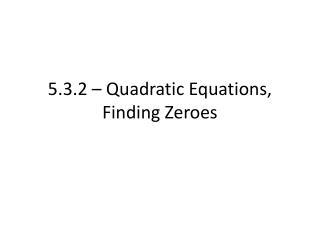DownloadDownload Presentation5.3.2 – Quadratic Equations, Finding Zeroes

# 5.3.2 – Quadratic Equations, Finding Zeroes

Télécharger la présentation## 5.3.2 – Quadratic Equations, Finding Zeroes

- - - - - - - - - - - - - - - - - - - - - - - - - - - E N D - - - - - - - - - - - - - - - - - - - - - - - - - - -
##### Presentation Transcript

1. 5.3.2 – Quadratic Equations, Finding Zeroes

2. Recall, we went over how to factor quadratics that are trinomials • Example. Factor the expression x2 + 7x + 12

3. Quadratic Equations • Quadratic Equations are written in the form ax2 + bx + c = 0 • If you can factor the left side, then you can solve the equation with a specific property • Known as finding the “zeroes,” or where the equation cross the x-axis

4. Zero Product Property • Zero Product Property = if A and B are expressions, then; • If AB = 0, then A = 0 OR B = 0 • In other words, if we can factor into two expressions, we can set each expression equal to zero

5. To solve quadratic equations; • 1) Factor the equation on the left side (factors of last that add to the middle) • 2) Write as a product of two binomials • 3) Use the zero product property, set each factor equal to zero • 4) Solve the one step equation • 5) Check by plugging numbers back into factored form

6. Example. Solve the quadratic equation x2 + 5x + 6 = 0 • Factors? • Zero Product Property?

7. Example. Solve the quadratic equation x2 - 3x - 28 = 0 • Factors? • Zero Product Property?

8. Example. Solve the quadratic equation x2 + 10x - 16 = 0 • Factors? • Zero Product Property?

9. Using Your Calculator • Using your graphing calculator, we can also find the zeroes, or x-intercepts, of quadratics

10. Assignment • Pg. 237 • 34-45 all